# 2018 CCPC 桂林赛区小结 By SmartLy @ Reconquista

• TYPE: Onsite Contest
• NAME: 2018 - CCPC - Guilin
• PLAT: HUSTOJ
• TIME: 2018/10/28 09:00-14:00 CST
• LOCA: Guilin University Of Electronic Technology
• TEAM: Reconquista [lsmll, jiangshibiao, lzw4896s]
• RANK: 3/188 1.60% (Including unofficial teams)
• SOLVE: 7/12
• PENALTY: 877
◦ A - (-3)
◦ B - 231
◦ C - 147 (+2)
◦ D - 30
◦ G - 20
◦ H - 22 (+2)
◦ J - 66
◦ L - 259 (+1)

## day-1

$$12:20$$ 的飞机飞桂林。飞多了有点无感了o(╯□╰)o……
第一次来桂林耶！可惜不能偷偷出去旅游┭┮﹏┭┮。中途还换了个酒店，就来不及报道了。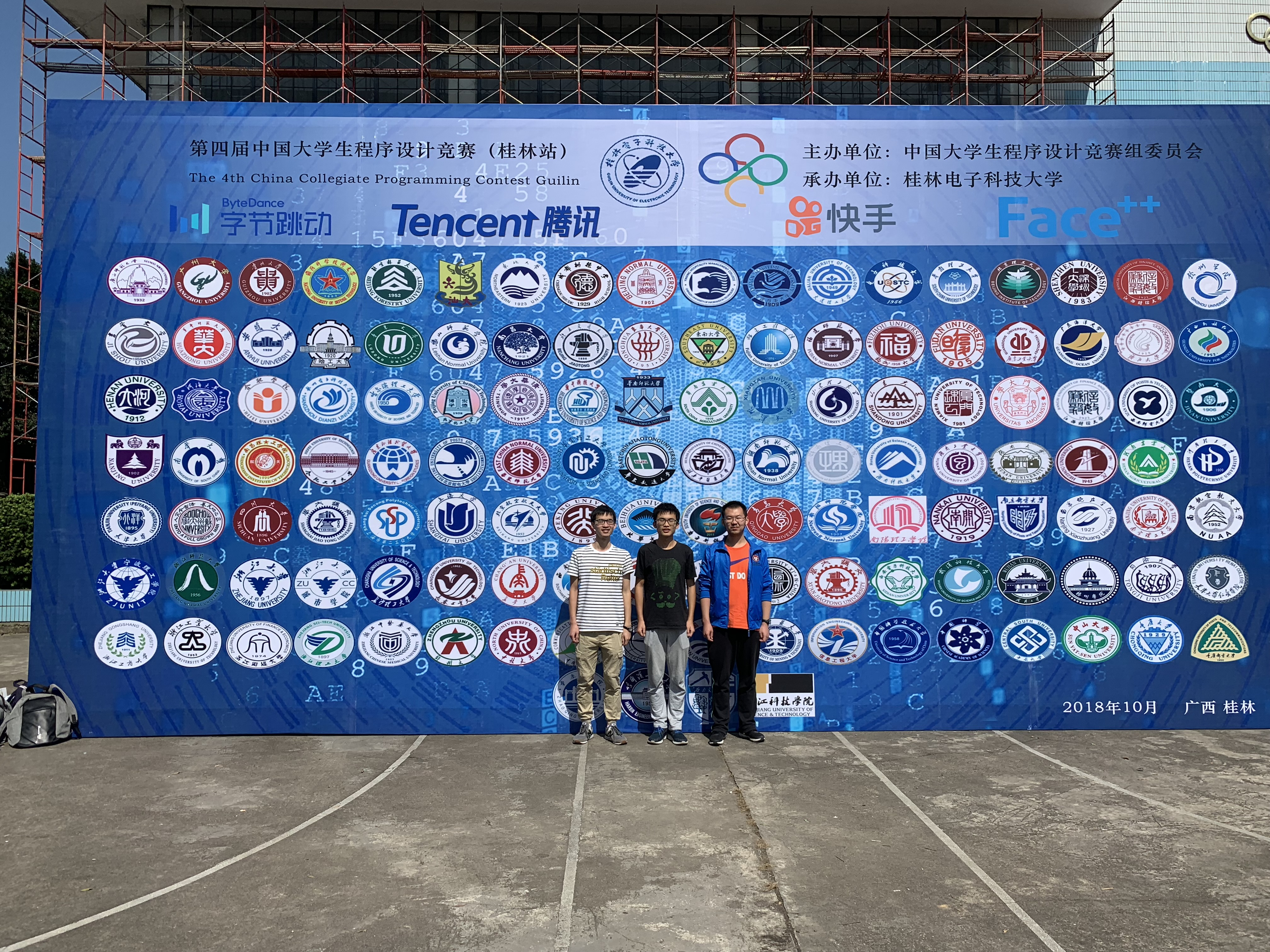比赛场地位于桂电的老校区，周围一圈全是小店铺和酒店。 $$lsmll$$ 学长觉得路边的店不太靠谱，于是我们分批打车去万达吃晚饭。
犹豫了半天吃啥。最后一半人去吃必胜客，我、欧阳和张鑫愉快地去烤鱼(#.#)。学习 $$EuropeSun$$ 沈阳的操作，饭后集体去电玩城 QAQ。不太适应这种嘈杂的环境，花花绿绿的不知道玩啥：我就在那儿疯狂投篮，投得手好酸呀！还记得和威威一起玩了喷水枪的游戏，但第二关就没过 o(╥﹏╥)o。哎呀啥时候去学学跳舞机，感觉很酷 (≧∇≦)ﾉ。
回程打车，我们花了 $$16$$，张鑫它们花了 $$42.6$$。哈哈哈竟然还是打表的，这出租车怕是改装过的吧 (#.#)。
实名举报张鑫，连续几天都在宾馆和女票视频，一聊就聊很久，吃饱狗粮 (╥╯^╰╥)。

## day0

起床迟了没吃到宾馆的早餐呜呜呜。
报道完就要吃中饭了，食堂的菜感觉不太行啊。$$15$$ 的饭票竟然只能换两瓶饮料，主办方在知乎被喷傻了。

然后就是下午的热身赛。原来队伍 $$id$$$$team96$$，可是我们的账号密码一直对不上；一个小时候，我们获得了 $$sorry96$$ 的账号——在排行榜上感觉特别霸气hhhh。题目质量还行吧， $$C$$ 题看起来要解微分方程，推了一会我就跑了。$$B$$ 题要写一个 $$py$$ 解释器，果断丢给了颜学长；后来发现还要高精度，我就上机手写了一个带负数的高精类，写得欲仙欲死。WA了几发之后竟然过了！大家都懒得写这题，所以最后排名挺好看的。

报道的时候看到了凯妹，在食堂吃中饭时看到了卓男和凌神，开幕式的时候看到了奕臻，到比赛场地的时候看到了飞鱼和金浩男。晚上拖着他们去万达小聚。选了火锅自助，大家疯狂拿肥宅快乐水，吃得很开心。竟然不能无限量拿羊肉，感觉血亏。本来想请客的，摸了摸口袋还是 $$AA$$ 算了……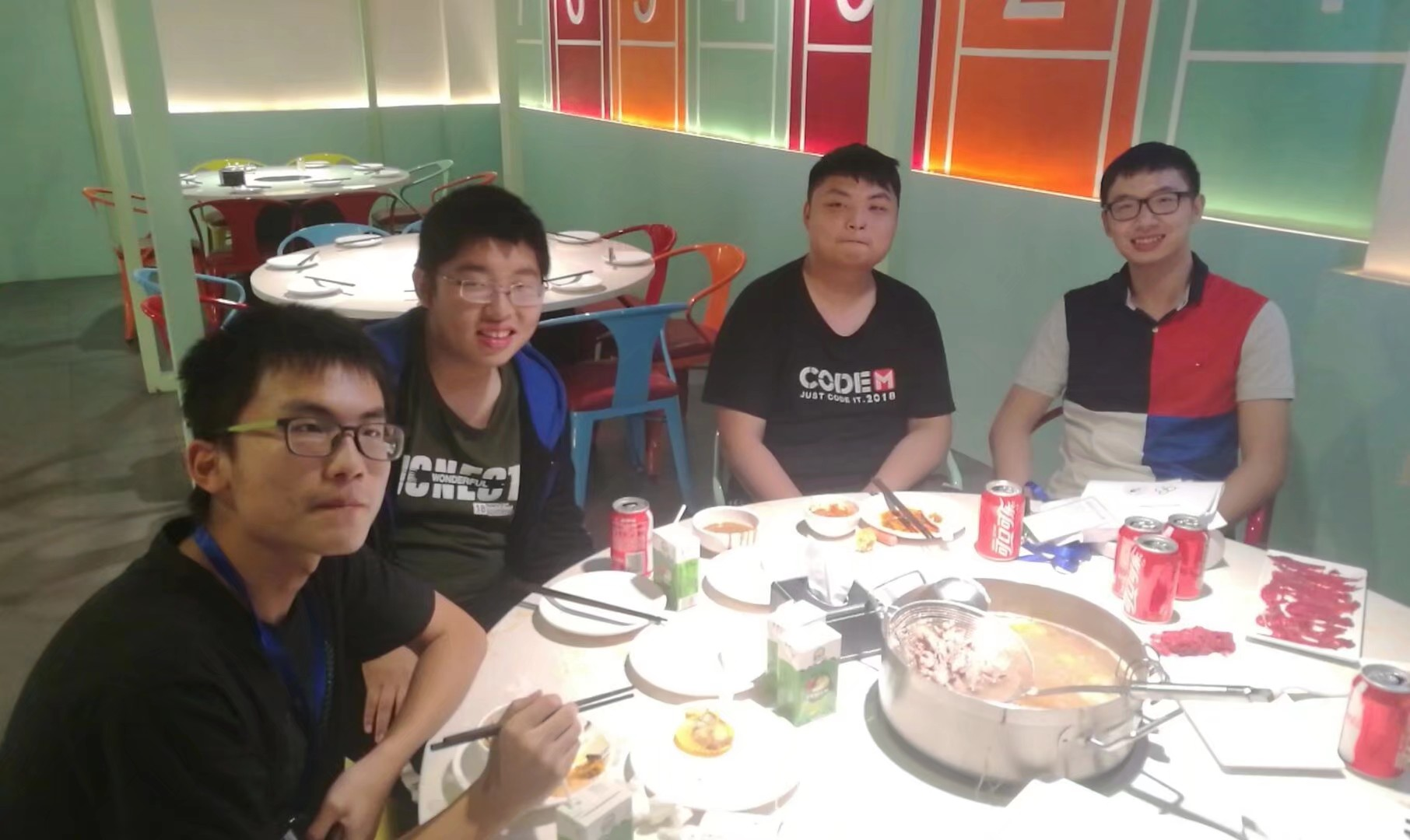晚上开了简短的会议。因为是 CCPC，大家都挺轻松的，没啥压力。

## day1

开场后正常开题。有点兴奋，看到 $$H$$ 是个傻逼逐位确定，上机写了会喜获WA。同时 $$lzw$$ 也会了 $$G$$，可惜抢一血失败，G1y20。我陆续发现了两个
bug，心累，H3y22。随后 $$lsmll$$ 上机写 $$D$$D1y30
这之后稍有点卡，榜上清华 $$13min$$ 就过了 $$A$$，我们想入非非，但随便想了几种贪心都是错的；$$J$$ 题的博弈一时冷静不下来想，打了个表也没啥规律。后来 $$lzw$$ 提出，$$J$$ 的最终方案是确定的！我脑补了一个相对大小不变的结论，J1y66。暂时放了放 $$A$$$$lsmll$$ 感觉 $$C$$ 比较可做，和我讨论了一会感觉会了，但是细节好多呀。此时清华过了 $$C$$，我就丢给了颜学长。$$C$$写的比较久，中途还 $$fix$$ 了几个细节，但是提交后 WA 了，我心态有点崩。这中间我向 $$lzw$$ 大致确认了 $$B$$$$NTT$$ 的做法，并决定单开 $$B$$，把 $$J$$ 的大讨论几何丢给 $$lzw$$$$C$$ 题我都差点想放弃了，不过一路 $$fix$$ 后竟然神奇地通过了，C3y147，仰慕颜学长。
之后 $$lsmll$$$$lzw$$ 合力搞 $$J$$，我开 $$B$$，我们轮流上机写题。$$B$$ 题我是在暴力的基础上一边改一边拍的，加了好几个 $$NTT$$ 后成功了，B1y231。他们俩 $$L$$ 写完发现过不了样例，一度怀疑是板子错了。我去帮忙看了看，发现有一个隐蔽的括号抄错了。过样例提交后还是没过，有点慌。好在随后 $$lzw$$ 发现有一种情况没有考虑，改了改，L2y259。然后就三个人集体攻 $$A$$，最后还是没有想出正确做法，交了几发乱搞意料之中地没过。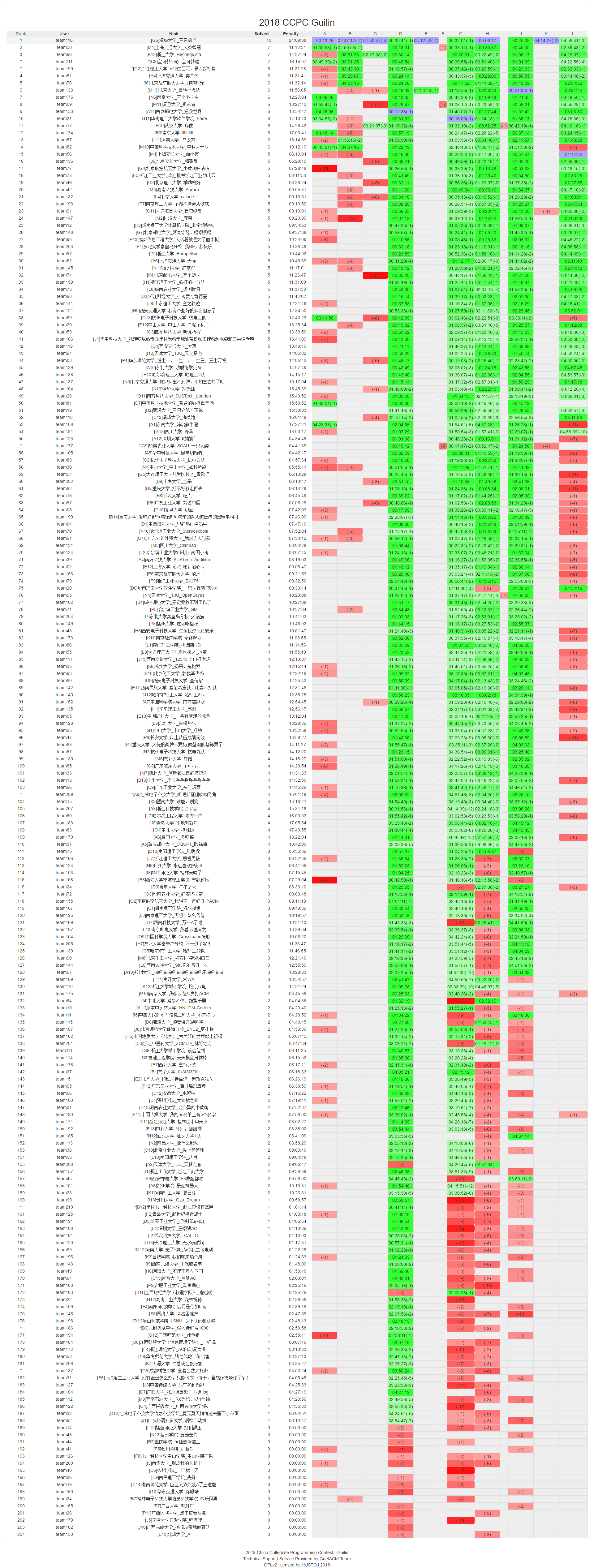有滚榜呢！不过滚得太快了，根本来不及看。好像别的队伍都挺弱？发挥平平还混了个季军。奖杯好大呀。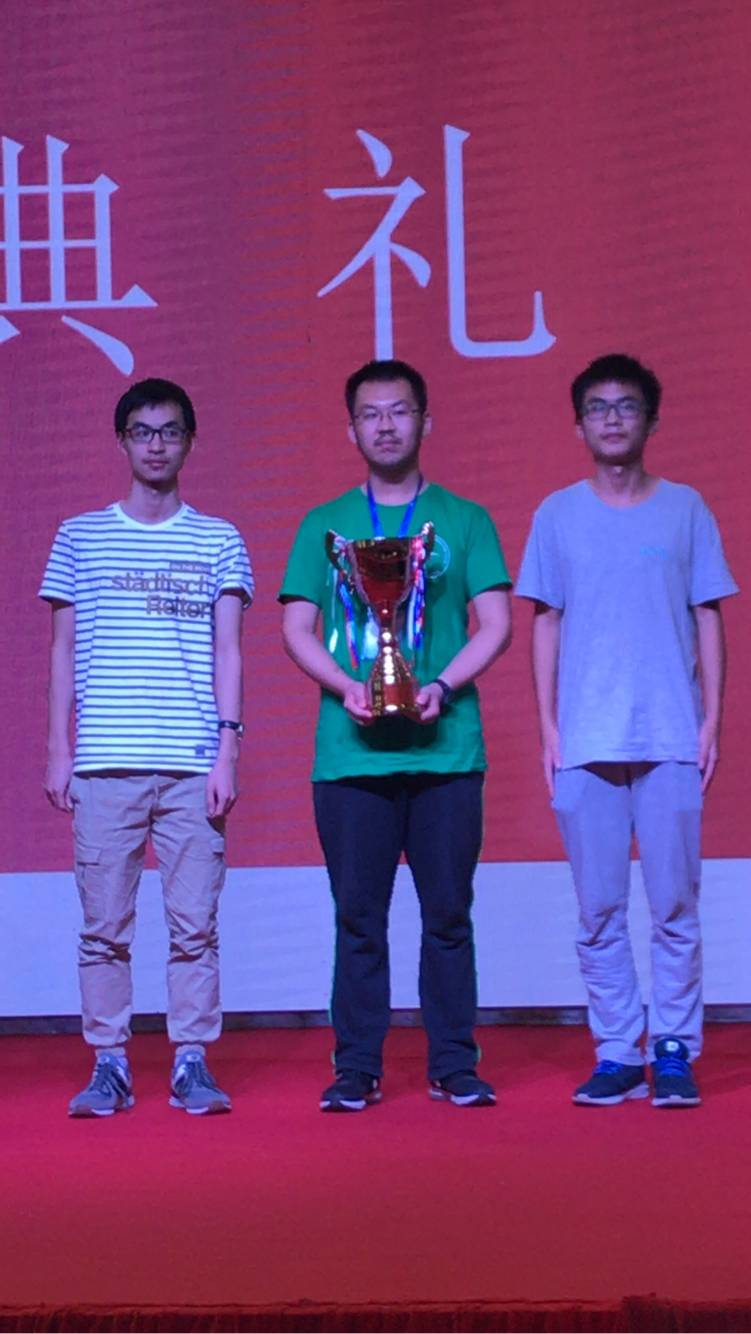晚饭在宾馆旁的饭店解决，算是我们队 $$bg$$ 吧。回房间发现 $$ds$$ $$project$$ 的截止时间是明天——于是倒头就睡。

## day2

多住了一晚。感觉张鑫小朋友睡得很迟啊，年轻真好。
回程要在南宁转机，轻松错过上午的课……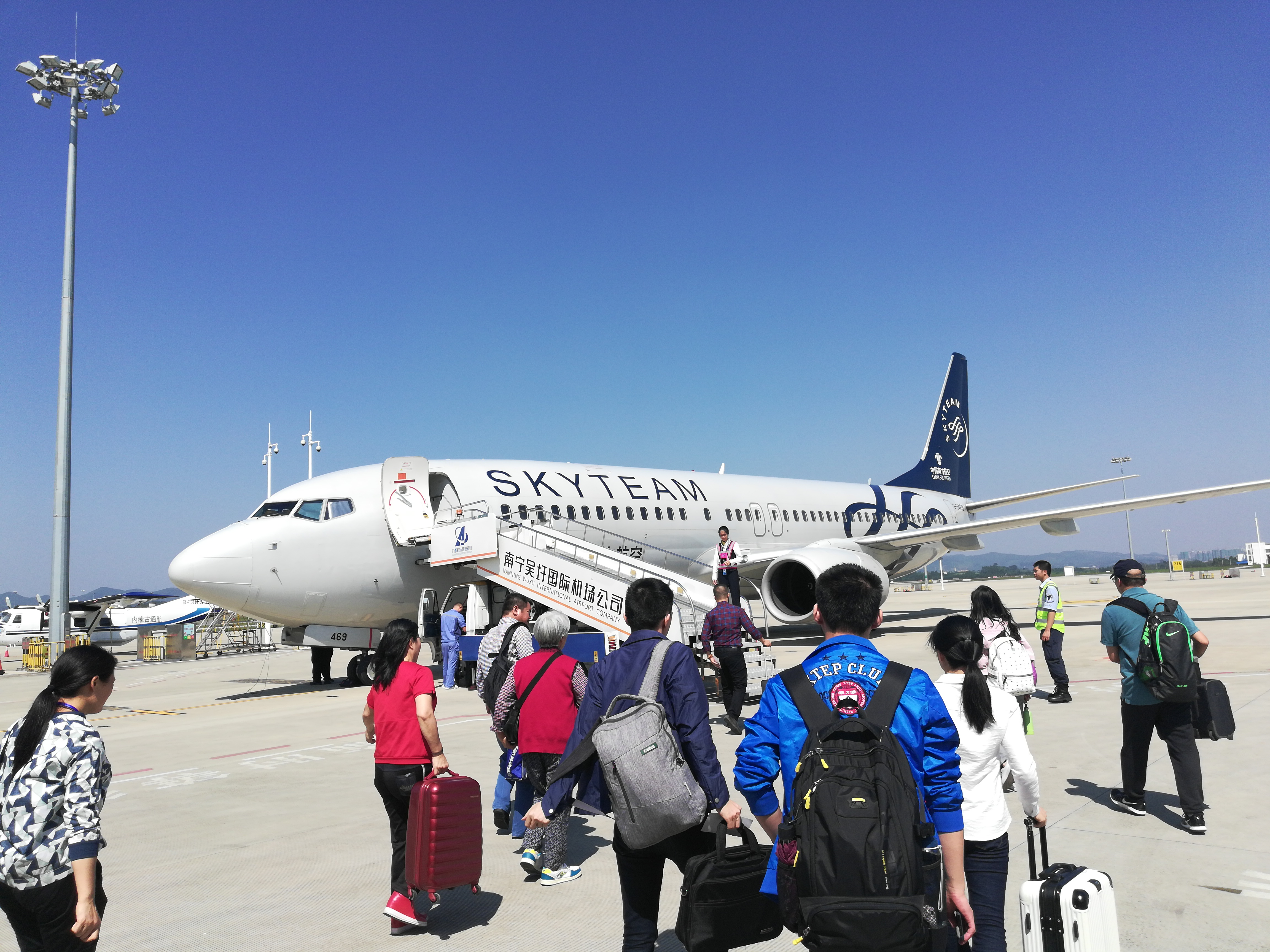## Summary

发挥平稳，没有失误也没有亮点，和南京差不多——屠版的 $$A$$ （当时是 $$B$$）不会做，但额外开了道 $$C$$ （当时是 $$L$$）。
当强队比较少的时候，我们就很容易被带榜。这次的 $$A$$ 其实挺智商的，而 $$E$$$$K$$ 如果去想一想，做出的可能性更大一点。
emmmm还是要多多磨合，多多锻炼吧！

posted @ 2018-10-30 12:52  了491  阅读(656)  评论(0编辑  收藏  举报